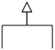# Create Generalization Set using Open APIThe generalization set defines a set of generalization relationship that describe how the superclass may be divided by a set of subclasses. The generalization defined in the set can group together in diagram for better presentation of the model. The generalization set can defined via the GUI on diagram or using Open API. In this article you will learn how to create generalization set using Open API in Visual Paradigm.

## Create Classes Diagram and Classes

Let’s start by create the class diagram and classes. We can use the DiagramManager.createDiagram() method to create the diagram.

```// Create Class Diagram
DiagramManager diagramManager = ApplicationManager.instance().getDiagramManager();
IClassDiagramUIModel diagram = (IClassDiagramUIModel) diagramManager.createDiagram(IDiagramTypeConstants.DIAGRAM_TYPE_CLASS_DIAGRAM);```

Once the diagram is created we then create class models and its view on diagram.

```// Create Super Class model and shape
IClass superClass = IModelElementFactory.instance().createClass();
superClass.setName("SuperClass");
IClassUIModel superClassShape = (IClassUIModel) diagramManager.createDiagramElement(diagram, superClass);
superClassShape.setBounds(150, 50, 80, 40);
superClassShape.setRequestResetCaption(true);```

Let’s try it yourself to create the sub-classes.

## Create Generalization

Once the classes are created we can then create the generalization model and connector.

```// Create generalization model and connector between super class and sub-classes
IGeneralization generalization1 = IModelElementFactory.instance().createGeneralization();
generalization1.setFrom(superClass);
generalization1.setTo(subClass1);
IGeneralizationUIModel generalization1Shape = (IGeneralizationUIModel) diagramManager.createConnector(
diagram, generalization1, superClassShape, subClass1Shape,
new Point[] {	new Point(190, 90),
new Point(190, 120),
new Point(90, 120),
new Point(90, 150)});
generalization1Shape.setRequestResetCaption(true);```

Now try it yourself to create the other generalization between superclass and subclass 2.

## Create Generalization Set

Now we can create generalization set model and associate it with the generalizations we created.

```// Create generalization set model
IGeneralizationSet generalizationSet = IModelElementFactory.instance().createGeneralizationSet();

// Add generalizations to generalization set

// Create generalization UI model
IGeneralizationSetUIModel generalizationSetUI = (IGeneralizationSetUIModel) diagramManager.createDiagramElement(diagram, generalizationSet);

// Set notation for generalization set to common arrow head

## Show up the diagram

Finally we can show up the diagram.

```// Show up the diagram
diagramManager.openDiagram(diagram);```

## Sample Plugin

The sample plugin attached in this article demonstrate how to create generalizations with generalization set. After you deploy the plugin into Visual Paradigm you can then click the plugin button in the application toolbar to trigger it.